edHelper.com Math Worksheets Time Worksheets 2nd Grade Telling TimeWorksheets, Lessons, and Printables

Telling Time Math Center (easier - hour hand does not move between numbers)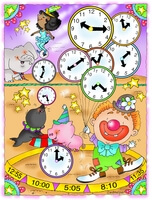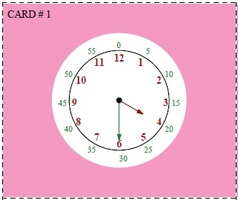Minutes shown on clock (Grades K-1) Normal clock - just hours shown on clock (Grades 1-3)

Telling Time Math Center (more difficult - hour hand moves with minutes like a real clock)Minutes shown on clock (Grades K-1) Normal clock - just hours shown on clock (Grades 1-3)

Telling Time Activity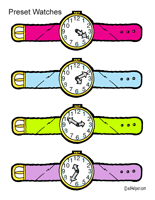Which Watch

Writing Numbers on a Clock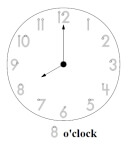Trace all the numbers on a clock

Book Units
Benjamin Banneker: Pioneering Scientist (Grades 2-5)

Missing Number on a Clock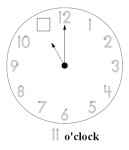Trace numbers on clock and fill in the missing number

Easy Clock and Time Matching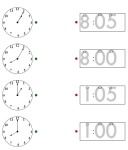Clock Matching: Hours Clock Matching: On the hour and five minutes past given (same hour used)

Hour Sequence:  Complete the Time Sequence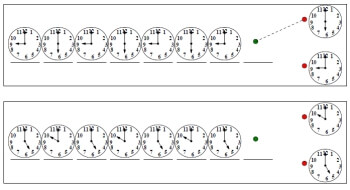Very easy clock sequence - First sequence has a trace answer line Very easy clock sequence - two different hours Very easy clock sequence - three different hours Two or three different hours in sequence More challenging clock hour sequence

Clock Activity Center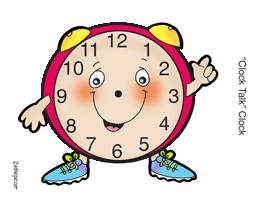Clock Talk

Hour
Write the time (____ o'clock; half past ____)
Write the time (____:____)
Write the time two ways
Show the time on the clock
Write the time two ways (smaller clocks)

Half-Hour
Write the time (half past ____)
Write the time (____:____)
Write the time two ways
Show the time on the clock
Write the time two ways (smaller clocks)

Mixed: Hour and Half-Hour (larger clocks)
Write the time (half past ____ and ____ o'clock)
Write the time (____:____)
Write the time two ways
Show the time on the clock

Mixed: Hour and Half-Hour (smaller clocks)
Write the time (half past ____ and ____ o'clock)
Write the time (____:____)
Write the time two ways
Show the time on the clock
Circle the correct time (2 choices)

Review
Telling Time Mixed Review

Leveled Books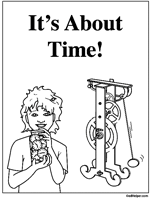It's About Time!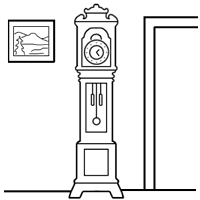My Grandmother's Grandfather Clock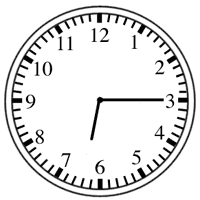What Time Is It?

Hour and Half-Hour
Write the time (____ o'clock)
Write the time (____:____)
Write the time two ways
Show the time on the clock
Circle the correct time
Write the time (other descriptions used)

Time to 15 Minutes
Write the time (____:____)
Show the time on the clock
Circle the correct time
Write the time (other descriptions used)

Time to 5 Minutes
Write the time (____:____)
Show the time on the clock
Circle the correct time
Write the time (other descriptions used)

Minutes
Write the time (____:____)
Show the time on the clock
Circle the correct time
Write the time (other descriptions used)

How long will it take? (estimating the time needed to do something)
Comparing things to do to one minute
Comparing things to do to 30 minutes
Mixed

Time Word Problems
Time to 10 minutes
Time to 5 minutes
Time to minutes

Time Patterns: Adding 5, 10, 15, 20, 25, and 30 minutes
Digital clocks are all given; need to draw hands on a few clocks
Analog clocks are all given; need to write the time
Complete the pattern

Time Patterns: Subtracting 5, 10, 15, 20, 25, and 30 minutes
Digital clocks are all given; need to draw hands on a few clocks
Analog clocks are all given; need to write the time
Complete the pattern

Time Patterns: Adding and subtracting 5, 10, 15, 20, 25, and 30 minutes
Digital clocks are all given; need to draw hands on a few clocks
Analog clocks are all given; need to write the time
Complete the pattern

Time Patterns (with smaller clocks): Adding and subtracting 5, 10, 15, 20, 25, and 30 minutes
Digital clocks are all given; need to draw hands on a few clocks
Analog clocks are all given; need to write the time
Complete the pattern

Time Patterns: Adding and subtracting 15, 30, 45, 60, 75, 90, 105, and 120 minutes
Digital clocks are all given; need to draw hands on a few clocks
Analog clocks are all given; need to write the time
Complete the pattern

Time Patterns: Adding and subtracting 1, 2, 3, 4, 5, 6, 7, 8, 9, and 10 minutes
Digital clocks are all given; need to draw hands on a few clocks
Analog clocks are all given; need to write the time
Complete the pattern

Understanding Time Words
Time Words (Half-Hour and Hour)
Time Words (Five Minutes)
Time Words (Any Time)
Time Words (Five Minutes; May Include "Quarter To")
Time Words (Any Time; May Include "Quarter To")

Telling Time

Have a suggestion or would like to leave feedback?